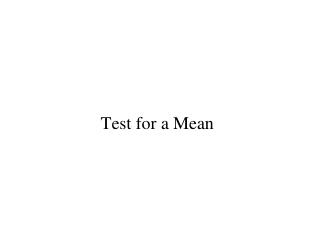DownloadDownload PresentationTest for a Mean

# Test for a Mean

Download Presentation## Test for a Mean

- - - - - - - - - - - - - - - - - - - - - - - - - - - E N D - - - - - - - - - - - - - - - - - - - - - - - - - - -
##### Presentation Transcript

1. Test for a Mean

2. Example A city needs \$32,000 in annual revenue from parking fees. Parking is free on weekends and holidays; there are 250 days in which parking is not free. This implies that the daily revenue must average \$128 or more over the long run. Officials initially set rates on the low end, hoping to attract more shoppers. After a trial period of 25 days, they will test the claim that too little revenue is being collected. The significance level is set at  = 0.05.

3. Test of a Mean H0:  = 128 H1:  < 128 Assuming sampling is done randomly: For a sample from a confirmed Normal population, or a sufficiently large sample, the test statistic t follows a(n approximate) t distribution with (n – 1) DF. • P-value computations depend on the orientation (right-, left- two-tailed) of H1.

4. Data 115.34 142.43 137.10 123.65 87.00 123.77 118.83 117.48 141.30 128.54 125.44 110.43 133.78 96.86 121.94 130.73 133.67 119.11 137.56 124.70 99.63 121.20 117.66 121.98 132.37

5. Example H0:  = 128

6. H0:  = 128 H1:  < 128 Test Statistic / P-value Since the test is left tailed, we need the area below -2.037 for a t distribution with 24 DF.

7. Sampling Distribution of T24 When H0 is True From Data From Table

8. Just a bit more than 0.025 Sampling Distribution of T24 When H0is True From Data From Table

9. Test Statistic / P-value Since the test is left tailed, we need the area below -2.037 for a t distribution with 24 DF. That area is between 0.025 and 0.05 and much closer to 0.025. Using Minitab we get: P-value = 0.026.

10. Corraboration T = -2.037 The critical value for a 5% test is –t0.05= -1.711. A 90% CI for : E = 1.711 2.61 = 4.47 118.0 <  < 127.0

11. Interpretation of P-value Suppose revenue has population mean \$128 per day. (Only) 2.6% of all possible samples of size 25 give a T statistic as low as the observed -2.037 (which comes from the sample mean of \$122.50, with standard deviation \$13.50).

12. Conclusion Since P-value = 0.026 < 0.05 we reject the null hypothesis. Simple, non technical interpretation of the conclusion… There is sufficient evidence in the data to conclude that the mean daily revenue is less than the needed \$128 per day.

13. Quiz – Based on Worksheet H0:  = 30 H1:  > 30 n = 19 DF = 18 T = 1.96 P-value = 0.033 True or false? 3.3% of the data is above 30. FALSE

14. Quiz H0:  = 30 H1:  > 30 n = 19 DF = 18 T = 1.96 P-value = 0.033 True or false? 3.3% of the data is below 30. FALSE

15. Quiz H0:  = 30 H1:  > 30 n = 19 DF = 18 T = 1.96 P-value = 0.033 True or false? 3.3% of the cars go 30. FALSE

16. Quiz H0:  = 30 H1:  > 30 n = 19 DF = 18 T = 1.96 P-value = 0.033 True or false? The probability the null hypothesis is true is 0.033. FALSE but if you think this way you’ll make correct decisions

17. What a P-value is… H0:  = 30 H1:  > 30 n = 19 DF = 18 T = 1.96 P-value = 0.033 Assume (pretend) the null hypothesis is true. Consider (pretend) the study is redone. The probability of a T at least as high as 1.96 is 0.033. T = 1.96 because the sample mean is 1.96 standard deviations above 30. Not so likely.